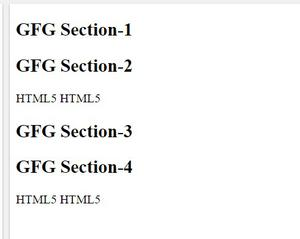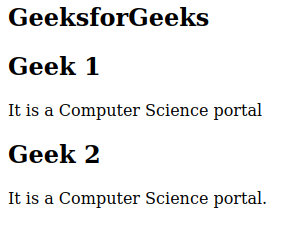Related Articles
How to define a section in a document in HTML5 ?
• Last Updated : 17 Mar, 2021

In this article we will discuss how to define section in document in html5.we can create section in document using Section tag.

HTML <section> Tag: Section tag divide content into section and subsection. The section tag is used when there is requriment of two header, footer and other section of document.

Syntax:

`<section> content </section>`

Below is the code that illustrates the use of the section tag.

Example 1:

## HTML

 ```<``html``>``<``body``>``  ``<``section``>``    ``<``h1``>GFG Section-1``  ```` ` `  ``<``section``>``    ``<``h1``>GFG Section-2``    ``<``p``> HTML5 HTML5``  ````  ``<``section``>``    ``<``h1``>GFG Section-3``  ```` ` `  ``<``section``>``    ``<``h1``>GFG Section-4``    ``<``p``>HTML5 HTML5``  `````` ` ``

Output:Example 2:

## HTML

 ```<``html``>``<``body``>``  ``<``h2``>GeeksforGeeks``  ``<``section``>``    ``<``h2``>Geek 1``    ``<``p``> ``      ``It is a Computer Science portal``    ```` ` `  ```` ` `  ``<``section``>``    ``<``h2``>Geek 2``    ``<``p``> ``      ``It is a Computer Science portal.``    ```` ` `  `````` ` ``

Output:My Personal Notes arrow_drop_up